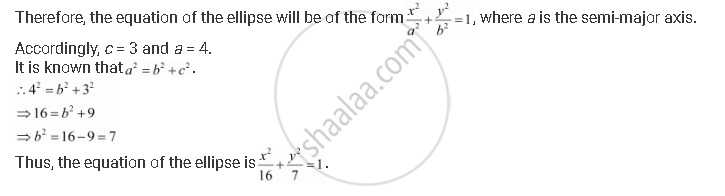# Find the Equation for the Ellipse that Satisfies the Given Conditions: Foci (±3, 0), a = 4 - Mathematics

Find the equation for the ellipse that satisfies the given conditions: Foci (±3, 0), a = 4

#### Solution

Foci (±3, 0), a = 4

Since the foci are on the x-axis, the major axis is along the x-axis.Concept: Ellipse - Standard Equations of an Ellipse
Is there an error in this question or solution?

#### APPEARS IN

NCERT Class 11 Mathematics
Chapter 11 Conic Sections
Q 17 | Page 255